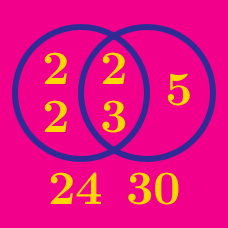Number Theory

# Greatest Common Divisor

What is the common divisor of $33$ and $55$ except $1$?

Simplify$\dfrac{2^2 \times 3}{2 \times 3 \times 5} .$

What is the greatest common divisor of 35 and 91?

What is the gcd(A, B) if:

A = $6 \times 35,$ and
B = $8 \times 15?$

Simplify $\dfrac{15}{20}.$

Details and assumptions:
- You may use the fact that $15= 3 \times 5$ and $20 = 4 \times 5$.

×